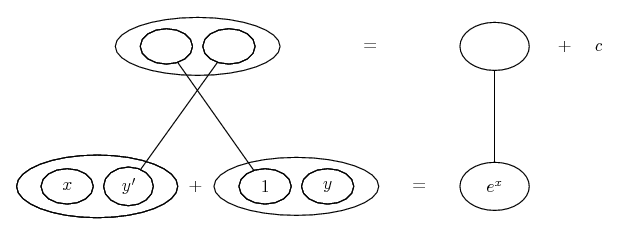# Thread: First order ordinary differential equation

1. ## First order ordinary differential equation

Solve the first order ordinary differential equation initial value problem for y(x):
x^2y'+xy=xe^x , y(1)=e+1

I have no idea even where to start.

Can anyone help please?

2. Divide through by x and the left hand side is 'exact', i.e. the evident 'result' of a product rule differentiation.

Edit:

Just in case a picture helps...... where... is the product rule. Straight continuous lines differentiate downwards (anti-differentiate up) with respect to x.

__________________________________________

Don't integrate - balloontegrate!

Balloon Calculus; standard integrals, derivatives and methods

Balloon Calculus Drawing with LaTeX and Asymptote!

3. Hopefully you at least recognize that this is a first order LINEAR differential equation; if it were seperable it would just be a matter of arranging the parts and gathering like terms. Remember that if we have a linear equation of the form $\displaystyle y'+P(x)y+Q(x)=0$, we can solve it using an integrating factor $\displaystyle \mu = e^{\int P(x)dx}$. We see we have y', multiplied by a function of x, and y multiplied by another function of x. Dividing through by x-squared:

$\displaystyle \frac{x^{2}y'}{x^{2}}+\frac{xy}{x^{2}}=\frac{xe^{x }}{x^{2}} \Rightarrow y'+\frac{y}{x}=\frac{e^{x}}{x}$

Now we have a linear differntial equation; our next step is to get an "integrating factor" (check your notes):

$\displaystyle \mu = e^{\int\frac{1}{x}}dx\Rightarrow \mu = e^{ln(x)} \Rightarrow \mu = x$

Multiplying both sides by the integrating factor:

$\displaystyle x(y'+\frac{y}{x})=x(\frac{e^{x}}{x}) \Rightarrow xy'+y=e^{x}$

It is here that you need to convince yourself that $\displaystyle xy'+y$, is just y times the integrating factor x, differentiated with respect to x (xy = y+xy'). Therefore we can write the left hand side as:

$\displaystyle (xy)'=e^{x}$

Integrating both sides:

$\displaystyle \int (xy)'=\int e^{x}dx \Rightarrow xy=e^{x}+C \Rightarrow y=\frac{e^{x}+C}{x}$

Now we just need to solve for C to get a specific solution to this differential, with x=1, and y=e+1:

$\displaystyle e+1=e^{1}+C \Rightarrow e-e+1=C \Rightarrow 1=C$

Therefore our final solution is

$\displaystyle y=y=\frac{e^{x}+1}{x}$

See if you can try this one using the exact same methods:

$\displaystyle y'=x^{3}-2xy; y(1)=2$

#### Search Tags

differential, equation, order, ordinary